# Multiplying by itself (squaring)

In mathematics, we call multiplying a number by itself squaring the number.

We call the result of squaring a whole number a square or a perfect square.

You should be able to fire out the answers to all squares up to 12times12

It’s just got to be inbuilt.

 2times2 = 4 3times3 = 9 4times4 = 16 5times5 = 25 6times6 = 36 7times7 = 49 8times8 = 64 9times9 = 81 10times10 = 100 11times11 = 121 12times12 = 144

## 4 x 4 = 16This 4x4 is a big machine I’m going to get one when I’m 16.

## 5 x 5 = 25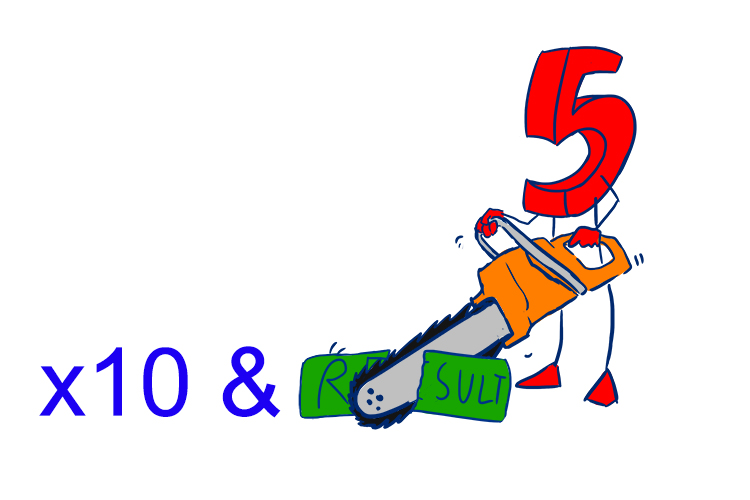Multiply by 10 and half the result.

## 6 x 6 = 36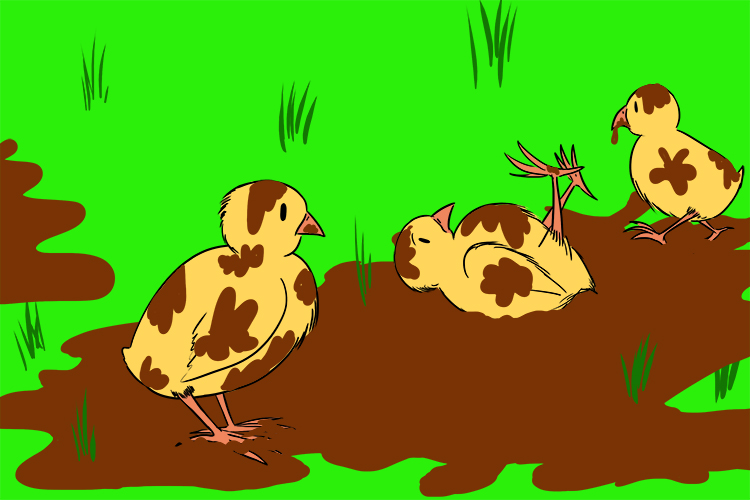Chicks, chicks, dirty chicks, six times six is thirty six.

## 7 x 7 = 49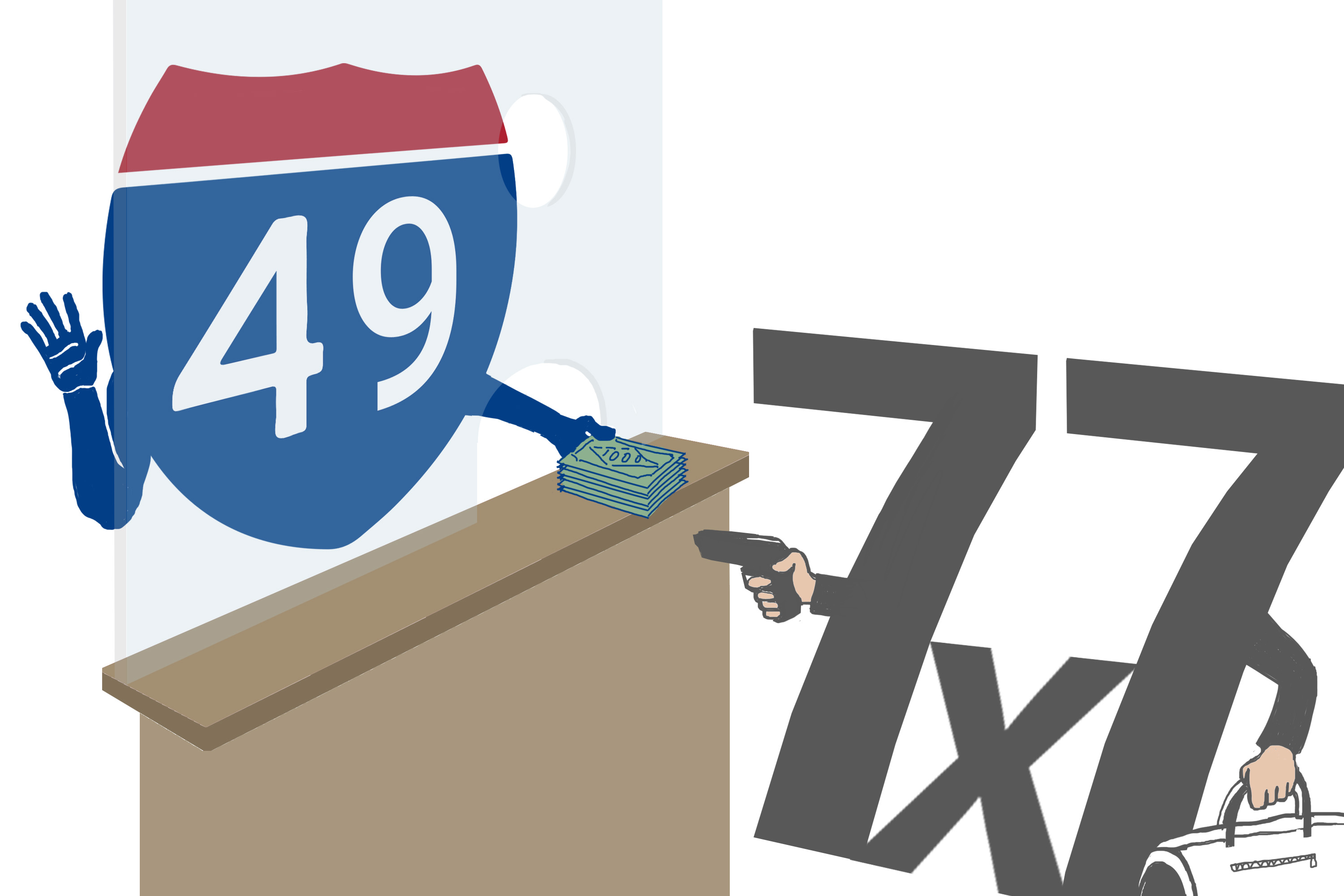Seven times seven commit a crime when they rob the number forty nine.

## 8 x 8 = 64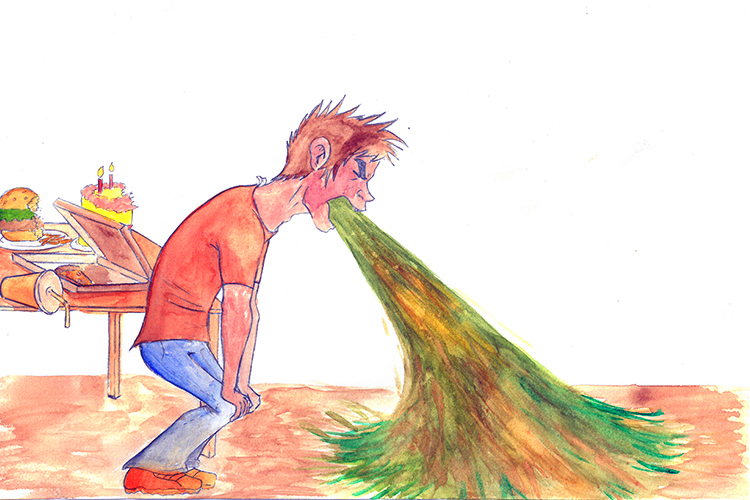I ate (8) and I ate (8) until I was sick (6) on the floor (4) equals sixty four.

## 9 x 9 = 81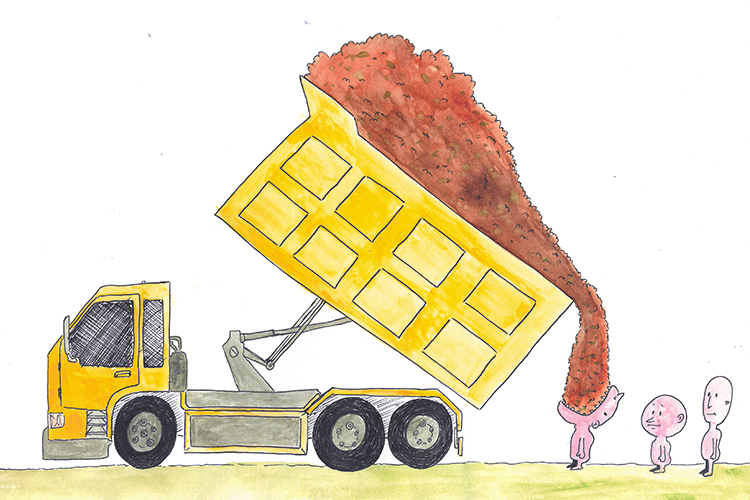He stood in line (9xx9) and ate one ton (81).

## 10 x 10 = 100Just put a zero on the end.

## 11 x 11 = 121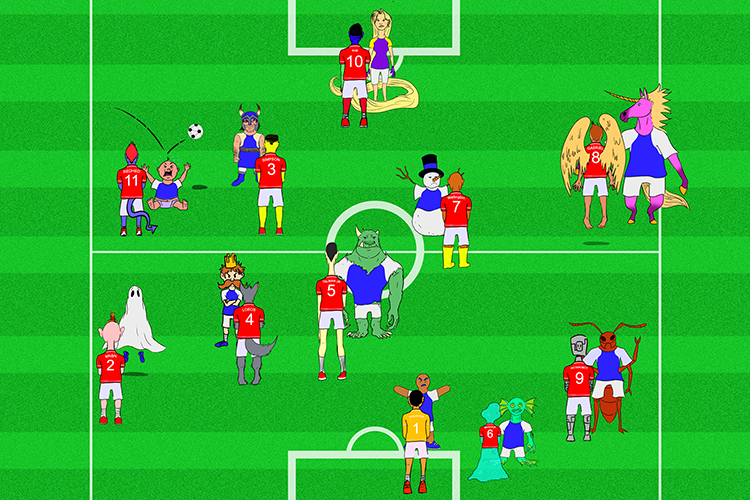The 11 players of the soccer team were told to mark the opposing 11 players one to one (121).

## 12 x 12 = 144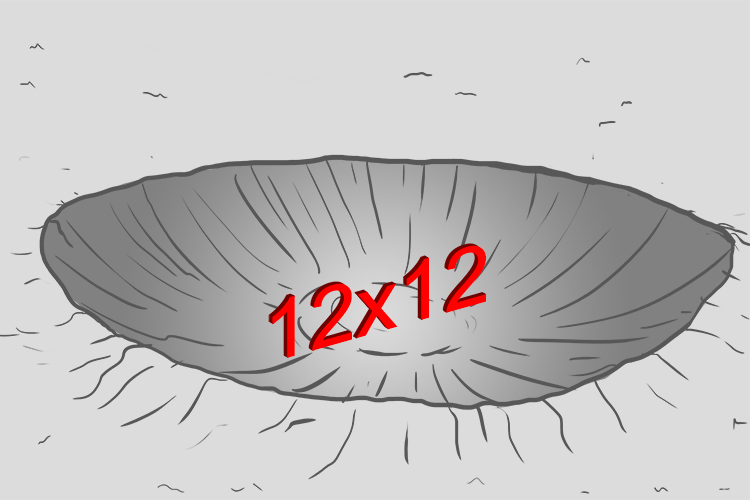12 times 12 is 144,

There is no more!